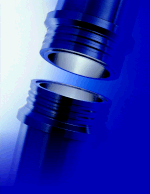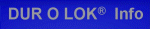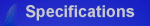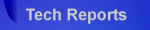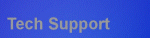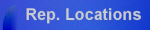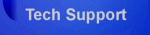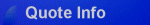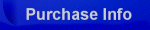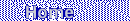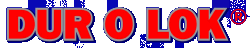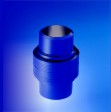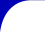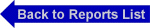THIS PAGE CONTAINS PROPRIETARY INFORMATION WHICH MUST NOT BE USED FOR COMPETITIVE PURPOSES OR IN ANY WAY DETRIMENTAL TO BETE FOG NOZZLE INC. ©BETE FOG NOZZLE, INC.

DESIGN OF COMPACT, HIGH PRESSURE
COUPLINGS AND CLOSURES
FOR
PRESSURE VESSELS AND PIPING
by
David H. Van Tassel, P.E.
And
Gifford L. Hitz

ABSTRACT

This paper presents the design and details of a pipe joint and closure for use in a wide variety of pressure vessel and piping applications including:  petrochemical, marine, nuclear, electric utility and synfuel, in low as well as high pressure.  The pipe joint consists of a pair of hubs locked together by a multi-grooved split coupler retained, in turn, by a tapered ring.

FIGURE 1            3 1500 lb ASA Flange vs. Equivalent 3 DUR O LOK pipe joint.

Comparison is made to a bolted flange demonstrating that the grooves direct forces through the coupling in a manner resulting in a substantially reduced level of stresses  effectively eliminating the causes of leakage in bolted flanges.  This method of load transmission further results in a compact coupling of significantly reduced weight and size, Figure 1, yielding numerous advantages over not only bolted flanges, but also, other couplings.

INTRODUCTION

Closures for pressure vessels and joints for piping introduce problems which are undoubtedly as old as the use of vessels and pipe.  Pressure vessel rules and flange standards have received regular review and revision down through the years.  However, most of the revisions in pressure vessel rules and the flange standards have centered around standardization of dimensions and calculations and updating material properties rather than conceptual improvements in joint design.

While in the majority of designs the practice of the past should be adequate (1), there exist admitted shortcomings in the conventional bolted flange.  These are evidenced not only by the discussion of such in Appendix S to Section VIII of the ASME Boiler and Pressure Vessel Code, but also by the various day-to-day experiences within industry.  As typical, when a synfuel pilot plant started full operation there were problems related primarily to pipe joints  the difficulties were described as leaks which made it impossible to maintain pressure in portions of the plant (2).

Troubles with bolted flanges are further demonstrated by design efforts over the years to devise connections which overcome their failure.  These efforts generally fall  into one of four types:

1)       Clamp

2)       Collet

3)       Shear Ring

4)       Shear Pin

and they utilize one of two methods of containment:

2)       Tapered rings or pins

The design effort by the authors is basically a clamp type coupling utilizing multiple shear members and a tapered ring, Figure 2.

Figure 2                   DUR O LOK pipe coupling.

DISCUSSION

The Problem

Having established that a problem exists with bolted flanges in some services, let us examine that problem and then, more importantly, its source in order that we might seek an orderly solution.

Leak.  The most popular connector used in industry is the bolted flange, however, in many applications the recurring problem is that they leak.  Granted, there are situations where this is nothing more than a minor inconvenience, but, certainly there are no services where a leak is desirable.

The leaking problem originates with the basic design of bolted flanges  the hydrostatic separating or end forces are transmitted through a circuitous path, Figures 3 and 4.  The resultant moments create bending stresses which require thick flanges and heavy hubs.  In turn, these

Figure 3         Moment Arm in 2-9/16 API Type 6BX

Welding Neck Bolted Flange

large material sections create metallurgical, thermal transient, handling (weight and space), operational and overall economic problems (3).  The moment increases rapidly for larger diameters and higher pressure, restricting the practical, leak-free use of this design.

Appendix S to Section VIII, Division 1 of the Code gives an excellent discussion of certain practical matters to be taken into consideration in order to obtain a serviceable design for bolted flange connections (1).  These considerations center around the bolts, the flanges and the gasket  the three components of a bolted flange connection.  Excessive stresses can be generated in any of these three components by any combination of three loads:  the initial bolt load, the hydrostatic end load generated by internal pressure and the load caused by thermal differentials.  Each of these is transmitted by all three components of the joint.

In the case of the bolts, excessive initial bolt load can introduce the problem of yielding in the bolting itself, which can occur in the tightening operation to the extent of damage or even breakage.  Any additional load generated when internal pressure is applied can produce further yielding with possible leakage.  An appreciable differential in temperature between the flanges and bolts or, a different coefficient of thermal expansion may cause an increase in bolt load which, when added to the load already existing, can result in yielding of the bolt.  Whereas, any pronounced decrease due to such effects can result in such loss of bolt load as to be a direct case of leakage.  In either case, retightening of the bolts may be necessary, but it must not be forgotten that the effects of repeated retightening can be cumulative and may ultimately make the joint unserviceable.

Excessive bolt load, whatever the reason, may cause the flange to yield, even though the bolts might not.  One of the most prevalent flange failures is irregular permanent distortion of the flange due to uneven bolt load around the circumference of the joint, causing the flange face and its gasket contact surface to warp out of a plane.  This yielding is due to the bending stress, Figure 3, usually the highest stress in the flange.

The gasket, too, can be overloaded, even without excessive bolt loads, because the full initial bolt load is imposed entirely on the gasket.  Accordingly, consideration must be given to prevent gross crushing of the gasket (1).

Figure 4         Moment Arm in 3 IPS SCH XXS

DUR O LOK Hub

Objectives

As the authors studied the problems encountered in vessel closures and pipe joints the following objectives were established are now realized:

-Design a smaller diameter joint to increase pipe rack utilization and simplify clearance problems.

-Design a lighter weight joint capable of matching full pipe strength.

-Utilize a true self energized seal as a simple replaceable element at each make-up of the joint.

-Eliminate bolt stretch and provide positive assembly not requiring special skill.

-Reduce the possibilities for jamming or galling of parts on disassembly.  Eliminate use of threads and super finished surfaces.

-Minimize the effects of uneven heating, rapid temperature change or pressure variation.

-Design a pipe joint in conformance to all applicable existing provisions of the code.

Means of Solution

As there are three components where problems with bolted flanges arise, the solution to the problem rests in affecting changes with these three.

First, the bolting is replaced by a multi-grooved split holding coupler.  The coupler replaces the possibility of the excessive initial bolt load as it has a predetermined level of stress defined by the geometry of the grooves.  The coupler also minimizes the possiblity of thermal loadings, due to the fact that it is in intimate contact with the hubs at the outside diameter of the piping as opposed to bolts in oversized holes at a significantly larger bolt circle.  In addition, the coupler has approximately twice the cross-sectional area of the bolts in joints of equal ratings, in most cases matching pipe strength, effectively reducing stress levels.  Aside from these improvements, the most significant advance is the reduction in the distance between the load reaction points; that is, the length of the bolting or coupler subject to elongation and relaxation

Figure 5       Distance Between Load Bearing Points in

a DUR O LOK Coupling

caused by the initial bolt load, hydrostatic end load and thermal load.  In even the most extreme services, this length in the coupler is a fraction of an inch, Figure 5; whereas, in the large high pressure bolted flanges this length exceeds one foot, Figure 6.

Second, the flanges are replaced by multi-grooved hubs.  The problem of the high bending moments in flanges is alleviated by arranging the load bearing surface in a multiplicity of levels similar to a gun breech lock.  This does not require a separate or special end forging, it can be machined directly in the cylinder wall and dismantling is a quick and simple opening and reclosing (4).  The high bending movements in bolted flanges are reduced to an insignificant level and the design basis becomes the more easily defined shearing and bearing stresses in the multiple grooves.

Finally, the typical gasket requiring a high seating stress is replaced by a true self energized gasket.  Two pressure energized gaskets are furnished:  One, a proprietary overcenter metal gasket for high temperature, critical or long-term service and the other, an O ring gasket for low temperature service.  These gaskets are easily replaced and obviate the need for carefully finished seats.  There is no danger of gross crushing or short-term relaxation of these gaskets  the initial seating is predetermined by the geometry of the grooves and the seal is maintained by internal pressure.  The exact defined and constant geometry of the gasket cavity makes all this possible.

THEORETICAL EVALUATION

In the calculation of bolted flange stresses, the moment of a load acting on the flange is the product of the load and its moment arm.  The moment arm is determined by the relative position of the bolt circle with respect to that of the load producing the moment (1), Figure 3.

Figure 6       Distance Between Load Bearing Points in

A 10 2500 lb. ASA Flange

As previously discussed, the design presented in this paper is directed toward significantly reducing these moments by minimizing the moment arms.  This is accomplished by locating the load bearing surfaces as nearly in alignment with the load as possible, Figure 4.  Accordingly, the coupler groove diameter is significantly less than the bolt circle diameter of an equivalent bolted flange.

Calculation of these moments yields an enlightening comparison between a bolted flange and the subject coupling.  The moments for a 2-9/16 inch API type 6BX Welding Neck Flange for 15,000 psi and the comparable 3 inch XXS Dur O Lok coupling were computed (Appendix).  These computations demonstrate that high bending moments, the major source of leaks in bolted flanges, have been significantly reduced by the coupling design; evidenced by a reduction in the total moment to approximately 7% that of the bolted flange, Table 1.

 2-9/16 API Type 6BX 3 IPS SCH XXS DUR O LOK Moment in-lb In-lb MD 157098 33512 MG 277270 0 MT 263823 12990 MO (operating) 698191 46502 MD (gasket seating) 647062 0

TABLE 1    Comparison of Moments in API Type 6BX

Flange and DUR O LOK

The fundamental design concept of the coupling presented in this paper is the reduction of stress levels and stress concentration.  In addition to the reduced stresses resulting from the reduced moments, the stresses are distributed more evenly.  The bolted flange shows increased stress levels at each bolt location, whereas, the design presented in this paper maintains a uniform stress level around the entire circumference of the coupling, without the possibility of mechanical differences in bolt and nut make up.

As a practical matter, axial shear stresses and bearing stresses are the stresses to be considered in designing the couplings.  By increasing the number of grooves provision can be made for additional loading.  Therefore, both the hub and the clamping couplers can be conservatively designed to accommodate high external loads in addition to pressure loads.

The most prevalent cause of leaking in bolted flanges, as pointed out in Appendix S of the ASME Code Section VII, Division 1, is the elongation of the bolts due to high initial bolt load, pressure increases or thermal cycle during service.  The elongation due to hydrostatic end force (pressure) is calculated (Appendix) according to conventional strength of materials theory, Table 2

 2-9/16 API Type 6BX 3 IPS SCH XXS DUR O LOK in. in. length subject to elongation 4.500 .355 elongation .01044 .00015

TABLE 2    Comparison of Elongation in API Type 6BX

Flange Bolts and DUR O LOK Couplers

While such elongation may appear small, a molecule of gas may be as small as .001 micron.  Thus, if a gasket takes a permanent set, gas can readily leak through a gap of .002 inch.  The coupling presented in this paper utilizes a pressure of self-energizing gasket which, together with an elongation 1/50 that of an equivalent bolted flange, operate to prevent leaks.

EMPIRICAL EVALUATION

During the past several years, 3 inch through 12 inch Dur O Lok couplings have been subjected to a variety of tests.  These test have been centered around a series conducted on 3 inch carbon steel couplings welded to 3 inch Schedule 80 ASTM A106 Grade B pipe.  The first of these tests was a high pressure test.  The pressure was cycled 50 times to 6,370 psi without any leakage, demonstrating lack of yielding due to relaxation and internal pressure.

The second was a steam test.  Steam pressure of 2,300 psi, generated by an external oxygen propane gas torch with direct flame impingement on one side of the coupling, was also cycled 5 times.  In neither case was there any leakage, demonstrating the resistance to yielding due to thermal effects.

The third test of the 3 inch SCH 80 carbon steel coupling was a bending test.  While internal pressure was maintained at 1,500 psi, an 8 foot assembly, with a hydraulic jack on the coupling in the middle and the ends supported, was deflected .45 inch without any leakage or distortion in the coupling, demonstrating a resistance to external loadings.

In addition, a 3 IPS XXS A105 carbon steel DUR O LOK pipe coupling utilizing a Viton elastometer O ring seal with an aluminum back-up ring and a one foot length of 3 IPS XXS A106 Grade B carbon steel pipe and a 3 IPS XXS A105 carbon steel weld cap welded to each end of the coupling has been subjected to cyclic testing and, finally, a design proof test as outlined in ANSI B16.9-1978.  This test assembly was cycled 5 times to 15,000 psi and held.  The pressure was then increased to 20,000 psi and held; then increased to 22,500 psi (the hydro test pressure for 15,000 psi design service) and held; then increased to 24,500 psi at which point the seal failed.  A new seal was placed in the coupling and the same procedure was repeated, hoever, in this second series the final pressure attained was 25,000 psi.  This test clearly demonstrates the design is well suited to high pressure applications  other of the authors seal designs allow this same 3 inch coupling to be used at more extreme pressures.

CONCLUSION

The coupling and closure presented in this paper offer designers important advantages over bolted flanged joints and blinds.  Not only do this coupling and closure eliminate leaks  the bolted joints greatest failing; they further provide a more compact and significantly lighter joint, they make up and break down in substantially less time in any position without bolt hole orientation.

The most important feature of this design is that all of these advantages are realized as a result of one of the most basic engineering principles  design improvement through minimized stresses and elimination of stress concentration.

REFERENCES

 1. ASME BOILER AN DPRESSURE VESSEL CODE, Section VIII, Division 1, 1980. 3. Pechacek, R., High Pressure, Quick Acting Closure for Large Diameter, Full Opening, Nuclear and Petro-Chem Pressure Vessels, ASME 78-PVP-74. 2.  SYNFUELS, McGraw-Hill, August 21, 1981. 4.  Jorgensen, S. M., Designs for Closures and Shell Joints, MECHANICAL ENGINEERING, Vol. 91, No. 6,

APPENDIX

For ease of comparison, calculations of the moments in a 2-9/16 API Type 6BX Welding Neck Flange for 15,000 psi maximum working pressure are made in the left column and calculations of the moments in a 3 XXS DUR O LOK Coupling at 15,000 psi are made in the right column.

 Figure A-1  2-9/16   API Type 6BX Welding Neck Flange Figure A-2   3 IPS SCH XXS DUR O LOK Hub

Ab = cross-sectional area of bolts, sq in

Ab = 8 (.606) = 4.848 in2                                        (1)

Am = total required cross-sectional area of bolts (couplers)

taken as the greater of Am1 and Am2, sq in

Am1 = 4.821 in2                                                       (2)

Am1 = total cross-sectional area of bolts (couplers)

required for operating conditions, sq in

Am1 = Wm1/Sb                                                         (3)

= 337471/70000 = 4.821 in2

Am2 = total cross-sectional area of bolts (couplers)

required for gasket seating, sq in

Am2 = Wm2/Sb                                                         (4)

= 15787/70000 = .226 in2

B = inside diameter of flange (coupling), in

B = 2.563 in                                                            (5)

b = effective joint-contact surface seating width, in

b = w/8 = .554/8 = .069 in                                      (6)

Ab = cross-sectional area of couplers, sq in

Ab = .475 p  (3.975) = 5.932 in2                             (1)

Am = 3.172 in2                                                        (2)

Am1 = Wm1/Sb                                                         (3)

= 79293/25000 = 3.172 in2

Am2 = Wm2/Sa                                                         (4)

= 0 in2

B = 2.300 in                                                            (5)

b = 0  self energized                                              (6)

C = bolt-circle (coupler) diameter, in

C = 7.875 in                                                            (7)

G = 4.046 in                                                            (8)

g1 = thickness of hub at back fo flange (coupling), in

g1 = 1.250 in                                                            (9)

H = total hydrostatic end force, lb

H = 0.785 G2P                                                        (10)

= 0.785 (4.046)2(15000) = 192758 lb

HD = hydrostatic end force on area inside of

flange (coupling), lb

HD = 0.785 B2P                                                      (11)

HG = W  H                                                            (12)

= 33741  192758 = 144713 lb

HP = total joint-contact surface compression load, lb

HP = 2b p Gm P                                                      (13)

= 2 p (.069)(4.046)(5.50)(15000) = 144713 lb

HT = difference between total hydrostatic end force and

the hydrostatic end force on area inside of flange

(coupling), lb

HT = H  HD                                                           (14)

= 192758  77350 = 115408 lb

hD = radial distance from the bolt (coupler) circle to the

circle on which HD acts, in

hD = R + g1/2                                                          (15)

=1.406 + 1.250/2 = 2.031 in

bolt (coupler) circle, in

hG = (C  G)/2                                                        (16)

= (7.875  4.046)/2 = 1.916 in

hT = radial distance from the bolt circle to the circle on

Which HT acts, in

HT = (R + g1 + hG)/2                                               (17)

MD = component of moment due to HD, in-lb

MD = HD hD                                                            (18)

= 77350(2.031) = 157098 in-lb

MG = HG hG                                                            (19)

+ 144713(1.916) = 277270 in-lb

MO = total moment acting on the flange (coupling), for

operating conditions or gasket seating as may

apply, in-lb

MO = MD + MG + Mr (operating)                         (20)

= 157098 + 277270 + 263823 = 698191 in-lb

C = 3.975 in                                                            (7)

G = 2.595 in                                                            (8)

g1 = .600 in                                                              (9)

H = 0.785 G2P                                                       (10)

= 0.0785 (2.595)2 (15000) = 79293 lb

HD = 0.785 B2P                                                     (11)

= 0.785 (2.300)2(15000) = 62290 lb

HG = W  H                                                            (12)

HP= 2bpGm P                                                         (13)

= 0 lb

HT = H  HD                                                           (14)

= 79293  62290 = 17003 lb

HD = R + g1/2                                                          (15)

= .238 + .600/2 = .538 in

hG = (C  G)/2                                                        (16)

= (3.975  2.595)/2 = .690

hT = (R + g1 + hG)/2                                                (17)

=(.238 + .600 + .690)/2 = .764 in

MD = HD hD                                                            (18)

=62290(.538) = 33512 in-lb

MG = HG hG                                                            (19)

= 0(.690) = 0 in-lb

MO = MD + MG + MT (operating)                         (20)

= 33512 + 0 + 12990 = 46502 in-lb

MO = W hG (assembly)                                          (21)

= 337715 (1.916) = 647062 in-lb

MT = component of moment du to HT, in-lb

MT = HT hT                                                           (22)

= 115408 (2.286) = 263823 in-lb

m = 5.50 (soft steel)                                               (23)

P = design pressure, psi

P = 15000 psi                                                         (24)

R = radical distance from bolt circle to point of intersection

of hub and back of flange, in

R = (C B)/2  g1                                                    (25)

= (7.7875  2.563)/2  1.250 = 1,362 in

Sa = Sb = allowable bolt (coupler) stress at ambient and

design temperature

Sa = Sb = 70000 psi (105000 psi min yield)           (26)

W = flange (coupling) design bolt (coupler) load, for the

operating condition or gasket seating, as may apply, lb

W = Wm1 (operating)                                             (27)

= 337471 lb

W = (Am + Ab)Sa/2 (gasket seating)                      (28)

= (4.821 + 4.828) 70000/2 = 337715 lb

Wm1 = minimum required bolt (coupler) load for the

operating conditions, lb

Wm1 = H + HP                                                        (29)

= 192758 + 144713 = 337471 lb

seating, lb

Wm2 = pbGy                                                            (30)

= p (.069) (4.046) (18,000) = 15787 lb

w = .554 in.                                                             (31)

y = 18000 psi                                                         (32)

in addition, bolt (coupler) elongation per classical strength of materials.

d = (FL) / (AE)                                                        (33)

F = Wm1 = 337471 lb                                              (34)

L = 4.500 in                                                            (35)

A = Ab = 4.848 in2                                                  (36)

E = 30 (10)6 psi                                                      (37)

d = (337471 x 4.500) / (4.848 x 30 x 106) = .01044 in

MO = W hG (assembly)                                           (21)

MT = HT hT                                                           (22)

= 17003 (.764) = 12990 in-lb

m = 0 (self energized)                                             (23)

P = 15000 psi                                                         (24)

R = (C  B)/2  g1                                                   (25)

= (3.975  2.300)/2 - .600 = .238 in

Sa = Sb = 25000 psi (105000 psi min yield)           (26)

W = Wm1 (operating)                                              (27)

= 79293 lb

W = 0 lb (self energized gasket)                              (28)

Wm1 = H + HP                                                        (29)

= 79293 + 0 = 79293 lb

= 0 lb

Wm2 = pbGy                                                            (30)

= 0 lb

w = 0 in. (self energized)                                        (31)

y = 0 psi                                                                 (32)

d = (FL) / (AE)                                                        (33)

F = Wm1 = 79293 lb                                              (34)

L = .355 in                                                              (35)

A = Ab = 5.932 in2                                                  (36)

E = 30 (10)6 psi                                                      (37)

d = (79293 x .355) / (5.932 x 30 x 106) = .00015 in

THIS PAGE CONTAINS PROPRIETARY INFORMATION WHICH MUST NOT BE USED FOR COMPETITIVE PURPOSES OR IN ANY WAY DETRIMENTAL TO BETE FOG NOZZLE INC. ©BETE FOG NOZZLE, INC.

Dur O Lok® manufactured by